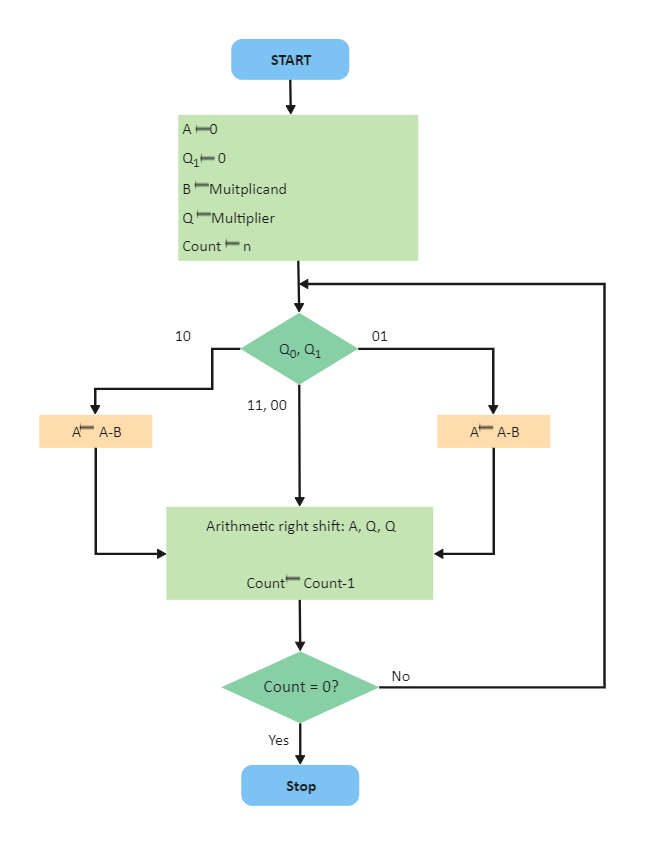Template Community / Booths Algorithm Flowchart

# Booths Algorithm FlowchartCharlotte
Published on 2021-07-16
Edit OnlineAs the below image illustrates, Booth's multiplication algorithm is a multiplication algorithm that multiplies two signed binary numbers in two's complement notation. It should be noted here that Booth Algorithm gives a procedure for multiplying binary integers in signed 2's complement representation inefficient way, i.e., less number of addition/subtractions required. The multiplicand is subtracted from the partial product upon encountering the first least significant 1 in a string of 1's in the multiplier. As the below algorithm suggests, the primary advantage of using the Booth Algorithm is that it handles both positive and negative multipliers uniformly. At the same time, it achieves efficiency in the number of additions required when the multiplier has a large block of 1's.
Tag
algorithm flowchart
Flowchart Collection
Report
0
242PostRecommended Templates